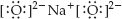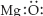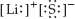×
Get Full Access to Introductory Chemistry - 5 Edition - Chapter 10 - Problem 44p
Get Full Access to Introductory Chemistry - 5 Edition - Chapter 10 - Problem 44p

×

# Determine what is wrong with each ionic Lewis structure and write the correctISBN: 9780321910295 34

## Solution for problem 44P Chapter 10

Introductory Chemistry | 5th Edition

• Textbook Solutions
• 2901 Step-by-step solutions solved by professors and subject experts
• Get 24/7 help from StudySoup virtual teaching assistantsIntroductory Chemistry | 5th Edition

4 5 1 418 Reviews
30
3
Problem 44P

PROBLEM 44P

Determine what is wrong with each ionic Lewis structure and write the correct structure.

(a)(b)(c)Step-by-Step Solution:
Step 1 of 3

Solution 44P

Here, we have to determine what is wrong with each ionic Lewis structure and write the correct structure.

(a):

We know, Na(Sodium) has 1 valence electron and O(Oxygen) has 6 valence electron. But oxygen has valency of 2. Hence, one O atom, need 2 Na atoms. Hence above lewis structure is incomplete. The correct lewis structure is :(b):

Mg(Magnesium) has 2 valence electron and O(Oxygen) has 6 valence electron. Oxidation state of Mg is 2+ and O is 2-. Hence, above lewis structure is incorrect. The correct lewis structure is :(c):

Li(Lithium) has 1 valence electron and S(Sulfur) has 6 valence electron. But S has oxidation from -2 to +6.  Hence, above lewis structure is incorrect. The correct lewis structure is:Step 2 of 3

Step 3 of 3

##### ISBN: 9780321910295

Unlock Textbook Solution